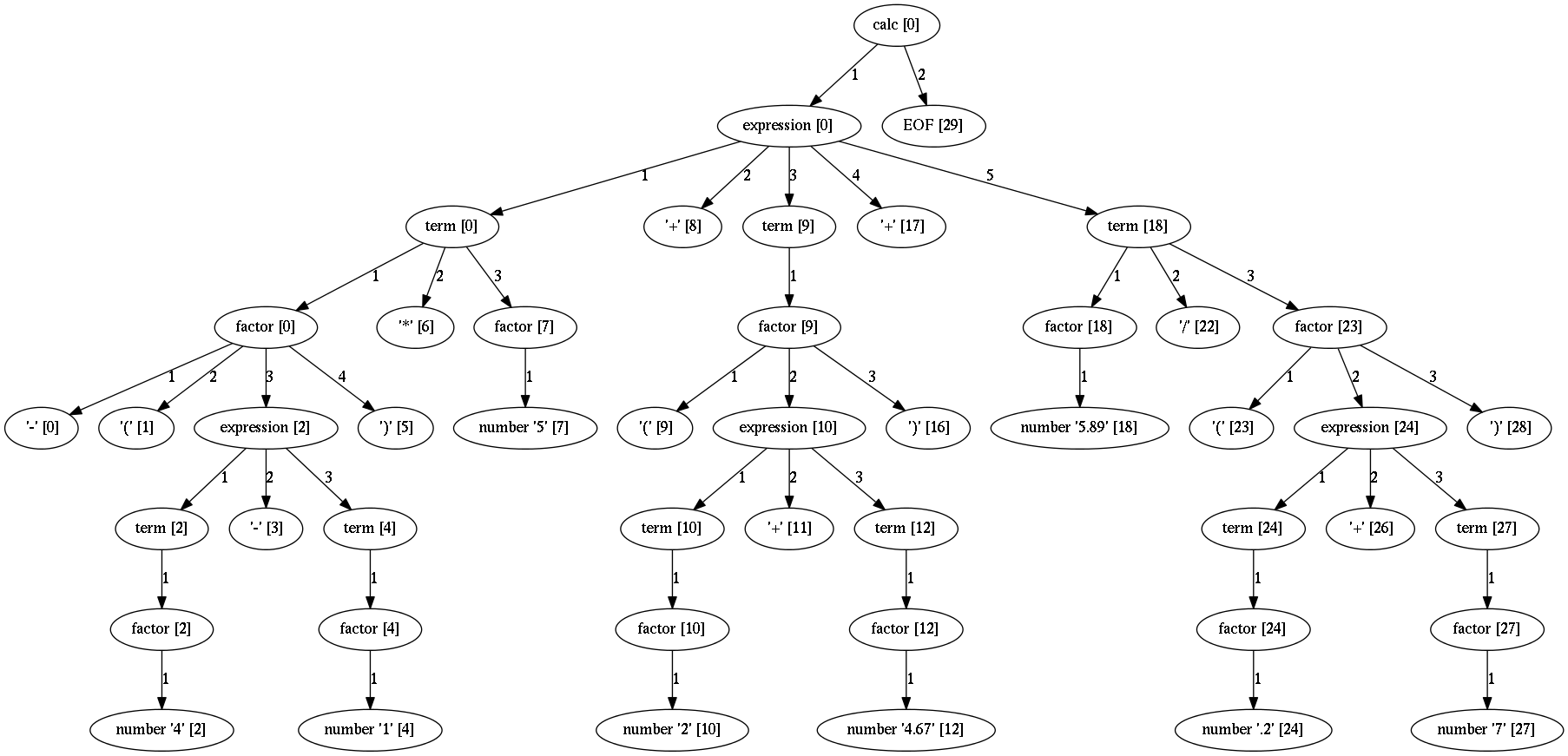# Calculator tutorial¶

A tutorial for parsing and evaluation of arithmetic expressions.

In this tutorial we will make a parser and evaluator for simple arithmetic expression (numbers and operations - addition, subtraction, multiplication and division). The parser will be able to recognize and evaluate following expressions:

``````2+7-3.6
3/(3-1)+45*2.17+8
4*12+5-4*(2-8)
...
``````

Evaluation will be done using support for semantic analysis.

Parsing infix expression has additional constraints related to operator precedence. Arpeggio is recursive-descent parser, parsing the input from left to right and doing a leftmost derivation. There is a simple technique that will enable proper evaluation in the context of a different operator precedence.

# The grammar¶

• Each `calc` file consists of one or more expressions.
``````def calc():       return OneOrMore(expression), EOF
``````
• Each expression is a sum or subtraction of terms.
``````def expression(): return term, ZeroOrMore(["+", "-"], term)
``````
• Each term is a multiplication or division of factors.
``````def term():       return factor, ZeroOrMore(["*","/"], factor)
``````

Note

Notice that the order of precedence is from lower to upper. The deeper is the grammar rule, the tighter is the bonding.

• Each factor is either a number or an expression inside brackets. The prefix sign is optional. This is a support for unary minus.
``````def factor():     return Optional(["+","-"]), [number, ("(", expression, ")")]
``````

Note

Notice indirect recursion here to `expression`. It is not left since the opening bracket must be found.

• And finally we define `number` using regular expression as
``````def number():     return _(r'\d*\.\d*|\d+')
``````

# The parser¶

Using above grammar specified in Python notation we instantiate the parser using `ParserPython` class.

``````  parser = ParserPython(calc)
``````

This parser is able to parse arithmetic expression like this

``````-(4-1)*5+(2+4.67)+5.89/(.2+7)
``````

and produce parse tree like thisNote

All tree images in this documentation are produced by running the parser in debug mode and using visualization support.

The parsing is done like this:

``````input_expr = "-(4-1)*5+(2+4.67)+5.89/(.2+7)"
parse_tree = parser.parse(input_expr)
``````

By ordering operation in the grammar form lower to upper precedence we have got the parse tree where the priority is retained. This will help us to easier make an expression evaluation.

# Evaluating parse tree¶

To implement evaluation we shall use Arpeggio's support for semantic analysis using visitor patter.

Visitor is an object with methods named `visit_<rule>` which gets called for each node of parse tree produced with the given rule. The processing of the tree nodes is done bottom-up.

``````class CalcVisitor(PTNodeVisitor):

def visit_number(self, node, children):
"""
Converts node value to float.
"""
return float(node.value)

...

``````

Visit method for the `number` rule will do the conversion of the matched text to `float` type. This nodes will always be the terminal nodes and will be evaluated first.

``````
def visit_factor(self, node, children):
"""
Applies a sign to the expression or number.
"""
if len(children) == 1:
return children
sign = -1 if children == '-' else 1
return sign * children[-1]

``````

Factor will have an optional sign as the first child and whatever matches first from the ordered choice of number and expression. We take the last element. It must be the result of `number` or `expression` evaluation and apply an optional sing on it.

Note

Note that the constant string matches will be removed by the Arpeggio, thus you will never get a constant string match in the children list.

``````
def visit_term(self, node, children):
"""
Divides or multiplies factors.
Factor nodes will be already evaluated.
"""
term = children
for i in range(2, len(children), 2):
if children[i-1] == "*":
term *= children[i]
else:
term /= children[i]
return term
``````

`term` consist of multiplication or divisions. Both operations are left associative so we shall run from left to right. Each even element will be evaluated `factor` while each odd element will be an operation to perform. At the end we return the evaluated `term`.

``````    def visit_expression(self, node, children):
"""
Term nodes will be already evaluated.
"""
expr = 0
start = 0
# Check for unary + or - operator
if text(children) in "+-":
start = 1

for i in range(start, len(children), 2):
if i and children[i - 1] == "-":
expr -= children[i]
else:
expr += children[i]

return expr
``````

And finally the whole expression consists of additions and subtractions of terms. A minor glitch here is a support for unary minus and plus sign.

Let's apply this visitor to our parse tree.

``````result = visit_parse_tree(parse_tree, CalcVisitor(debug=debug))
``````

The result will be a `float` which represent the value of the given expression.

# The grammar in PEG¶

As a final note, the same grammar can be specified in textual PEG syntax.

Either a clean PEG variant:

``````number = r'\d*\.\d*|\d+'
factor = ("+" / "-")?
(number / "(" expression ")")
term = factor (( "*" / "/") factor)*
expression = term (("+" / "-") term)*
calc = expression+ EOF

``````

``````number <- r'\d*\.\d*|\d+';